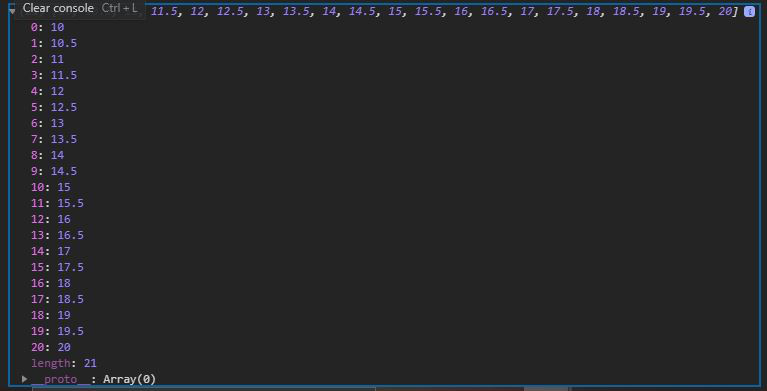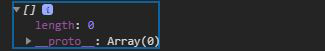# D3.js ticks() Function

• Last Updated : 19 Jul, 2020

The ticks()  function in D3.js is used to form an array of between a given range of start and stop both inclusive such that each element is uniformly and equally spaced.

Syntax:

```d3.ticks(start,stop,count);
```

Parameters: This function accepts three parameters as mentioned above and described below:

• start: It is the starting value from where we want the array element to be, it is inclusive.
• stop: It is the starting value to which we want the array element to be, it is inclusive.
• count: It is the number of elements we want in a given start and stops range.

Return value: It returns an array of nicely-rounded numbers.

Below given are a few examples of ticks() function.

Example 1:

## HTML

 ```<``html` `lang``=``"en"``>``<``head``>``  ``<``meta` `charset``=``"UTF-8"``>``  ``<``meta` `name``=``"viewport"` `        ``content``=``"width=device-width, initial-scale=1.0"``>``  ``<``title``>Document````<``body``>``  ````  ``<``script` `type` `= ``"text/javascript"` `          ``src` `= ``"https://d3js.org/d3.v4.min.js"``>``  ````  ``<``script``>``    ``// Choosing 10 elements in a range``    ``// Note that each element is equally spaced``    ``console.log(d3.ticks(10, 20, 20))``  ```````

Output:Example 2:

## HTML

 ```<``html` `lang``=``"en"``>``<``head``>``  ``<``meta` `charset``=``"UTF-8"``>``  ``<``meta` `name``=``"viewport"` `        ``content``=``"width=device-width, initial-scale=1.0"``>``  ``<``title``>Document````<``body``>``  ````  ``<``script` `type` `= ``"text/javascript"` `          ``src` `= ``"https://d3js.org/d3.v4.min.js"``>``  ````  ``<``script``>``    ``// Choosing 10 elements in a range``    ``console.log(d3.ticks(10, 20))``  ```````

Output:My Personal Notes arrow_drop_up